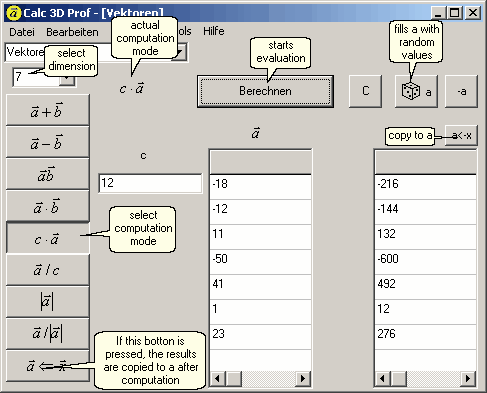There are 8 differently computation modes:
Addition, subtraction, multiplication (2 vectors, dot product, number with vector), length, unit vector.
For 3 dimensions other 3 computation modes are added:
Cross product, Spatprodukt, angle between 2 vectors.
The following illustration shows the mode, in which a number is multiplied by a 7-dimensionalen vector.